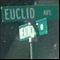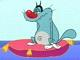# Math Is Fun Forum

Discussion about math, puzzles, games and fun.   Useful symbols: ÷ × ½ √ ∞ ≠ ≤ ≥ ≈ ⇒ ± ∈ Δ θ ∴ ∑ ∫  π  -¹ ² ³ °

You are not logged in.

## #1 2009-06-16 03:06:46

dentrillsfrac
Member
Registered: 2009-06-16
Posts: 4

### There are infinitely many primes p such that p + 2 is also prime.

There are infinitely many primes p such that p + 2 is also prime.

proof

We assume that the biggest pair is p and p+2. We can know create the function :

L(n,p)= n(1/p(p+2))

Where p+2 is the largest asummed prime number of the pair p and p+2
and  n is the a positive integer  greater than p+2.
The function L gives the number of integers that have as factor p and p+2(up to n).

If we suppose that there exist a larger pair of the form p+2k and p+2(k+1) Than the set of numbers that have as factors p+2k and  p+2(k+1) must be equal to 0 (since they do not exist).

L(n,p+2k)= n/((p+2k)(p+2(k+1))
Or
k-->oo
We supposed that p+2k and p+2(k+1) were integers. The numbers p+2k and p+2(k+1) does not exist unless L(n,p+2k) is not equal to 0 !! So there will always be a fraction of the set Z that will have p+2k and p+2(k+1)  in their unique prime factorization. So there is  an ifinite  number of primes p such that p + 2 is also prime.

please tell me is all wrong???

Offline

## #2 2009-06-16 03:22:53

bobbym
bumpkinFrom: Bumpkinland
Registered: 2009-04-12
Posts: 109,606

### Re: There are infinitely many primes p such that p + 2 is also prime.

Hi dentrillsfrac;

This is the twin prime conjecture a very old and hard problem in number theory.

In mathematics, you don't understand things. You just get used to them.
If it ain't broke, fix it until it is.
Always satisfy the Prime Directive of getting the right answer above all else.

Offline

## #3 2009-06-16 03:41:19

dentrillsfrac
Member
Registered: 2009-06-16
Posts: 4

### Re: There are infinitely many primes p such that p + 2 is also prime.

at the start i was very proud about this but i asked my bigger brother an he told me that i must be more serious!!!!!!:D

Last edited by dentrillsfrac (2009-06-16 03:42:15)

Offline

## #4 2009-06-16 03:53:01

dentrillsfrac
Member
Registered: 2009-06-16
Posts: 4

### Re: There are infinitely many primes p such that p + 2 is also prime.

please tell me that it is true!!!!

Offline

## #5 2009-06-16 04:00:45

bobbym
bumpkinFrom: Bumpkinland
Registered: 2009-04-12
Posts: 109,606

### Re: There are infinitely many primes p such that p + 2 is also prime.

Sorry, I can't spot the error in your logic. Please wait for some other opinions and don't be discouraged if your proof is not quite correct. This is one of the great unsolved problems in number theory since  Euclid's time (2300-2400 years ago).

Last edited by bobbym (2009-06-16 09:15:24)

In mathematics, you don't understand things. You just get used to them.
If it ain't broke, fix it until it is.
Always satisfy the Prime Directive of getting the right answer above all else.

Offline

## #6 2009-06-16 04:16:56

dentrillsfrac
Member
Registered: 2009-06-16
Posts: 4

### Re: There are infinitely many primes p such that p + 2 is also prime.

ok thank you anyway...!!!
make L that round to the closer integer ie if it is 1.2 the result should be 1!!!

Offline

## #7 2009-06-16 14:20:42

Ricky
ModeratorRegistered: 2005-12-04
Posts: 3,791

### Re: There are infinitely many primes p such that p + 2 is also prime.

I'm having a lot of trouble understanding what it is you're trying to go for.

If we suppose that there exist a larger pair of the form p+2k and p+2(k+1) Than the set of numbers that have as factors p+2k and  p+2(k+1) must be equal to 0 (since they do not exist).

We are supposing that there exists a larger pair where p+2k and p+2(k+1) are both prime?  This doesn't make sense, we've already supposed that p and p+2 were the largest pair with this property.

And you say that the number of integers that have p+2k and p+2(k+1) as factors must be zero.  This is false, just look at the number:

(p+2k)*(p+2(k+1))

This number has both those as a factor.

"In the real world, this would be a problem.  But in mathematics, we can just define a place where this problem doesn't exist.  So we'll go ahead and do that now..."

Offline

## #8 2009-06-16 22:16:25

Kurre
MemberRegistered: 2006-07-18
Posts: 280

### Re: There are infinitely many primes p such that p + 2 is also prime.

Ricky wrote:

I'm having a lot of trouble understanding what it is you're trying to go for.

If we suppose that there exist a larger pair of the form p+2k and p+2(k+1) Than the set of numbers that have as factors p+2k and  p+2(k+1) must be equal to 0 (since they do not exist).

We are supposing that there exists a larger pair where p+2k and p+2(k+1) are both prime?  This doesn't make sense, we've already supposed that p and p+2 were the largest pair with this property.

And you say that the number of integers that have p+2k and p+2(k+1) as factors must be zero.  This is false, just look at the number:

(p+2k)*(p+2(k+1))

This number has both those as a factor.

I guess he means that, because we assumed that p,p+2 where the largest pair there does not exist any numbers with factors p+2k,p+2(k+1). IM not sure though and I have not tried to understand the proof either.

Offline

## #9 2009-06-17 00:40:57

Ricky
ModeratorRegistered: 2005-12-04
Posts: 3,791

### Re: There are infinitely many primes p such that p + 2 is also prime.

I guess he means that, because we assumed that p,p+2 where the largest pair there does not exist any numbers with factors p+2k,p+2(k+1).

As I posted before, this statement is false.  (p+2k)*(p+2(k+1)) has precisely those numbers as factors.

"In the real world, this would be a problem.  But in mathematics, we can just define a place where this problem doesn't exist.  So we'll go ahead and do that now..."

Offline

## #10 2009-06-22 04:23:48

Kurre
MemberRegistered: 2006-07-18
Posts: 280

### Re: There are infinitely many primes p such that p + 2 is also prime.

Ricky wrote:

I guess he means that, because we assumed that p,p+2 where the largest pair there does not exist any numbers with factors p+2k,p+2(k+1).

As I posted before, this statement is false.  (p+2k)*(p+2(k+1)) has precisely those numbers as factors.

hm I meant prime factors, but maybe he does not use that they are prime factors, idk, im too lazy to read through the oproof atm.

Offline